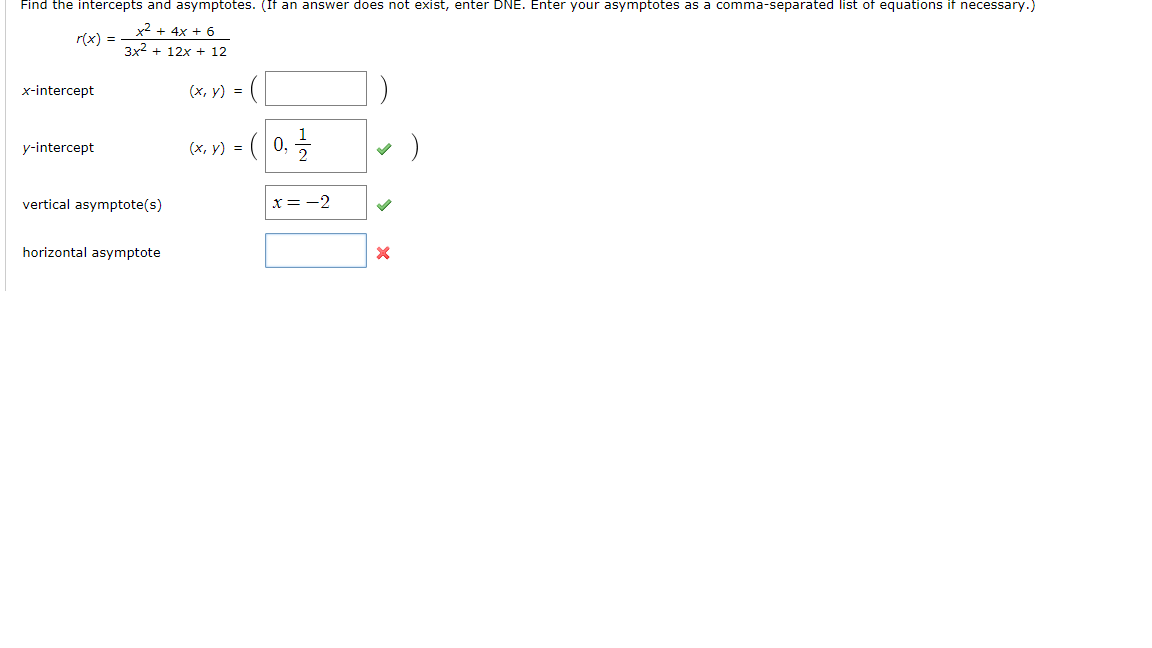# Find the intercepts and asymptotes. (If an answer does not exist, enter DNE. Enter your asymptotes as a comma-separated list of equations if necessary.)+4x+ 63x 12x + 12rx)x-intercept(x, y) =(x, y)(10, .2y-interceptvertical asymptote(s)horizontal asymptote

Question
11 views

Also need domain and rangehelp_outlineImage TranscriptioncloseFind the intercepts and asymptotes. (If an answer does not exist, enter DNE. Enter your asymptotes as a comma-separated list of equations if necessary.) +4x+ 6 3x 12x + 12 rx) x-intercept (x, y) = (x, y) (10, .2 y-intercept vertical asymptote(s) horizontal asymptote fullscreen
check_circle

Step 1

Given:

Step 2

Calculation:

x-intercepts:

Equate the given function to 0 and simplify.

Step 3

y-intercepts:

Substitute x = 0 in the...

### Want to see the full answer?

See Solution

#### Want to see this answer and more?

Solutions are written by subject experts who are available 24/7. Questions are typically answered within 1 hour.*

See Solution
*Response times may vary by subject and question.
Tagged in

### Algebra